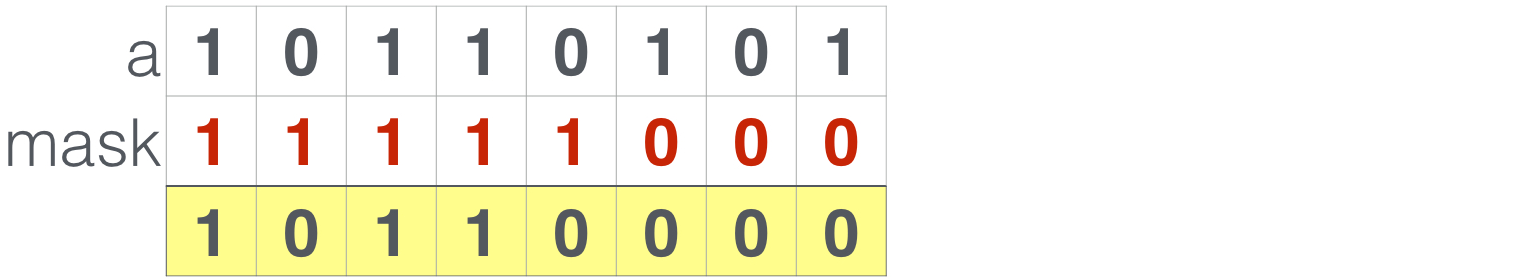# Solidity位操作运算符简介

Solidity位操作运算有助于缩减以太坊交易的成本。本文介绍以太坊智能合约开发语言 Solidity中的位操作运算符，以及如何使用这些Solidity位操作符对合约数据执行位操作运算，例如与、或、非、异或等，同时也介绍如何实现Soldity不支持的取反、移位等操作。

## 1、Solidity位操作概述``````bytes1 a = 0xb5; //  
bytes1 b = 0x56; //  ``````

## 2、与运算/AND``````
a & b; // 结果: 0x14  
``````

## 3、或运算/OR``a | b; // 结果: 0xf7    ``

## 4、异或运算/XOR``a ^ b; // 结果: 0xe3    ``

``0xe3 ^ a; // 结果: 0x56 == b    ``

## 5、非运算/NEGSolidity本身并不支持非运算，不过幸运的是你可以将变量与全1值 异或，就得到同样的结果：

``````
a ^ 0xff; // Result: 0x4a  
``````

## 6、移位运算/SHIFT

``00001230  ``

``01230000  ``

``00000123  ``

### 6.1 左移位``````var n = 3;
var aInt = uint8(a); // Converting bytes1 into 8 bit integer
var shifted = aInt * 2 ** n;
bytes1(shifted);     // Back to bytes. Result: 0xa8  
``````

### 6.2 右移位``````var n = 2;
var aInt = uint8(a); // Converting bytes1 into 8 bit integer
var shifted = aInt / 2 ** n;
bytes1(shifted);     // Back to bytes. Result: 0x2d  
``````

## 7、提取前N位``````var n = 5;
var nOnes = bytes1(2 ** n - 1); // Creates 5 1s
var mask = shiftLeft(nOnes, 8 - n); // Shift left by 3 positions
a & mask; // Result: 0xb0    ``````

## 8、提取后N位

``````var n = 5;
var lastBits = uint8(a) % 2 ** n;
bytes1(lastBits); // Result: 0x15    ``````

## 9、数据压缩``````bytes1 c = 0x0d;
bytes1 d = 0x07;
var result = shiftLeft(c, 4) | d; // 0xd7   ``````

## 完整源代码

``````
contract BitsAndPieces {
function and(bytes1 a, bytes1 b) returns (bytes1) {
return a & b;
}

function or(bytes1 a, bytes1 b) returns (bytes1) {
return a | b;
}

function xor(bytes1 a, bytes1 b) returns (bytes1) {
return a ^ b;
}

function negate(bytes1 a) returns (bytes1) {
return a ^ allOnes();
}

function shiftLeft(bytes1 a, uint8 n) returns (bytes1) {
var shifted = uint8(a) * 2 ** n;
return bytes1(shifted);
}

function shiftRight(bytes1 a, uint8 n) returns (bytes1) {
var shifted = uint8(a) / 2 ** n;
return bytes1(shifted);
}

function getFirstN(bytes1 a, uint8 n) returns (bytes1) {
var nOnes = bytes1(2 ** n - 1);
var mask = shiftLeft(nOnes, 8 - n); // Total 8 bits

}

function getLastN(bytes1 a, uint8 n) returns (bytes1) {
var lastN = uint8(a) % 2 ** n;
return bytes1(lastN);
}

// Sets all bits to 1

function allOnes() returns (bytes1) {
return bytes1(-1); // 0 - 1, since data type is unsigned, this results in all 1s.
}

// Get bit value at position
function getBit(bytes1 a, uint8 n) returns (bool) {
return a & shiftLeft(0x01, n) != 0;
}

// Set bit value at position
function setBit(bytes1 a, uint8 n) returns (bytes1) {
return a | shiftLeft(0x01, n);
}

// Set the bit into state "false"
function clearBit(bytes1 a, uint8 n) returns (bytes1) {
}
}  ``````

• 发表于 2020-02-04 00:14
• 阅读 ( 2541 )
• 学分 ( 20 )
• 分类：Solidity